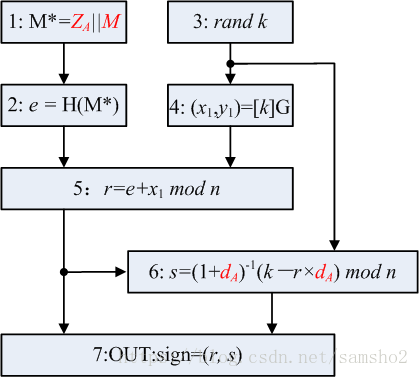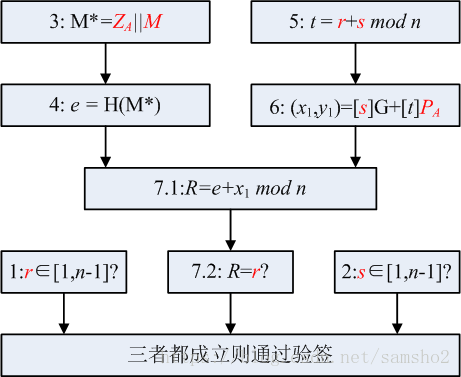## sm2签名过程

1. 计算 $M^*=Z_A |M$
2. 计算 $e=H_v(M^*)$，按规则将e的数据类型转换为整数；
3. 产生随机数$k \in [1,n-1]$
4. 计算椭圆曲线点$(x_1,y_1)=KG$，按规则将$x_1$的数据类型转换为整数；
5. 计算 $r=(e+x_1)\ mod\ n$，若r = 0或 r + k = n则返回3；
6. 计算 $s=((1+d_A)^{-1} (k-r d_A))\ mod\ n$，若 s = 0则返回3；
7. 按规则将r、s的数据类型转换为字节串，得到消息M的签名为(r,s)。 [注：按sm2的曲线参数，则r和s分别为32字节，即签名值为64字节]

0x31 0x32 0x33 0x34 0x35 0x36 0x37 0x38 0x31 0x32 0x33 0x34 0x35 0x36 0x37 0x38## sm2验证签名

1. 检验 $r \in [1,n-1]$，若不成立则验证不通过；
2. 检验 $s \in [1,n-1]$是否成立，不成立当然也不通过
3. 计算 $M^* =Z_A | M$；
4. 计算$e = H_v(M^∗)$，按规则将e的数据类型转换为整数；
5. 计算$t = (r + s) mod\ n$，若t = 0，则验证不通过；
6. 计算椭圆曲线点$(x_1,y_1)= [s]G +[t]P_A$
7. 按规则将$x_1$的数据类型转换为整数，计算$R = (e + x_1) mod\ n$，检验 R = r 是否成立，若成立则验证通过；否则验证不通过。

[注：$H_v$表示消息摘要长度为v比特的杂凑算法或者哈希算法，没什么特别之处] 流程图可表示如下：## 小结

• 发表于 2020-08-20 16:59
• 阅读 ( 551 )
• 学分 ( 8 )
• 分类：入门/理论